# 数据科学多元统计学 02：几何观点和高维数据

## 墨尔本大学 MAST90138 课程笔记

Posted by YEY on August 11, 2020

# Lecture 02 几何观点和高维数据

• Hardle, W. and Simar, L (2015). Applied multivariate statistical analysis, 4th edition.
• Hastie, T. Tibshirani, R. and Friedman, J. (2009). The elements of statistical learning, 2nd edition

## 1. 几何观点

### 1.1 距离

• 两个向量 $x,y\in \mathbb R^p$ 之间的 欧几里得距离 (Euclidian distance) $d(x,y)$ 被定义为：

$d(x,y)=\sqrt{\sum_{i=1}^{p}(x_i-y_i)^2}=\sqrt{(x-y)^{\mathrm T}(x-y)}$

二维空间 $\mathbb R^2$ 中的例子，其中 $x=(x_1,x_2)$，$y=(y_1,y_2)$：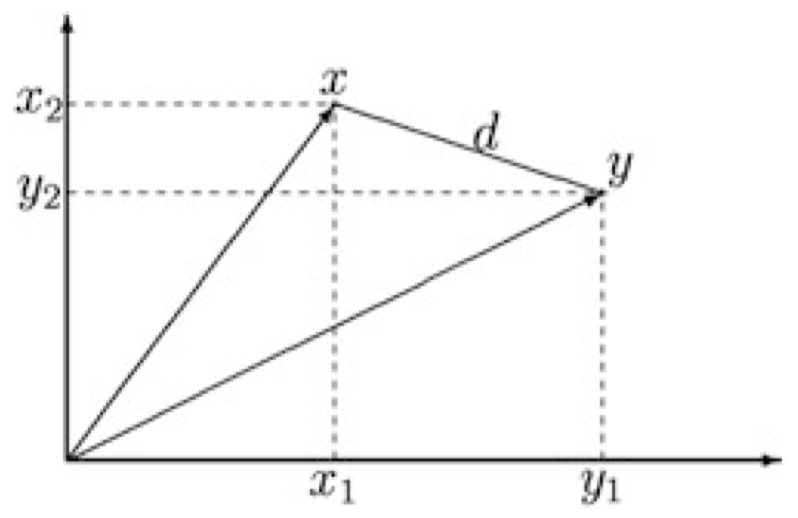图 1：距离 $d$

• 该距离的一个 加权版本 可以被定义为：

$d(x,y)=\sqrt{\sum_{i=1}^{p}w_i(x_i-y_i)^2}=\sqrt{(x-y)^{\mathrm T}\mathcal W(x-y)}$

其中，每个 $w_i>0$，并且 $\mathcal W=\mathrm{diag}(w_1,\dots,w_p)$。

• 这可以被进一步推广到下面的距离：

$d(x,y)=\sqrt{(x-y)^{\mathrm T}\mathcal A(x-y)}$

其中，$\mathcal A$ 是一个 正定 矩阵。

### 1.2 范数

• 一个向量 $x\in \mathbb R^p$ 的 (欧几里得) 范数 (Norm) 被定义为：

$\|x\|=\sqrt{\sum_{i=1}^{p}x_i^2}=\sqrt{x^{\mathrm T}x}$

• 一个 单位向量 是一个范数为 $1$ 的向量。

• 可以推广到关于一个 正定 矩阵 $\mathcal A$ 的范数：

$\|x\|_{\mathcal A}=\sqrt{x^{\mathrm T}\mathcal A x}$

### 1.3 两个向量的夹角

• 两个向量 $x,y\in \mathbb R^p$ 之间的夹角 $\theta$ 由 $\theta$ 的余弦值定义：

$\cos(\theta)=\dfrac{x^{\mathrm T}y}{\|x\|\|y\|}$

在二维空间 $\mathbb R^2$ 中的例子：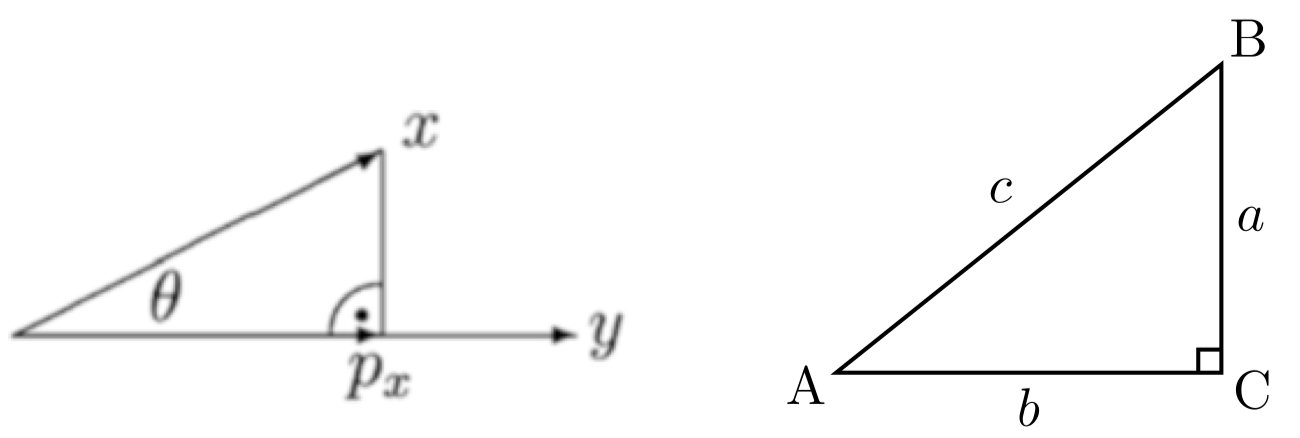图 2：向量夹角和投影

• 根据三角学我们知道，在角 $C$ 为直角的直角三角形 $ABC$ 中，角 $A$ 的余弦值等于 $AC$ 段的长度 $b$ 除以 $AB$ 段的长度 $c$，因此

$\cos(\theta)=\dfrac{p_x}{\|x\|}$

其中，$p_x$ 被称为 $x$ 在 $y$ 上的 投影 (Projection)。回归之前的两个向量夹角的余弦定义，我们可以计算出 $p_x$ 为：

$p_x=\cos(\theta)\|x\|=\dfrac{x^{\mathrm T}y}{\|y\|}$

### 1.4 旋转

• 对于 $p$ 维空间 $\mathbb R^p$ 中的向量，我们通常使用 $p$ 轴系统 来描述它们，并给出 $x$ 在该 $p$ 坐标系中的坐标。

• 在多元统计学中，有时将轴 (所有轴同时) 旋转 一个角度 $\theta$ 会很有用，可以通过这种方式创建一个新的 $p$ 轴坐标系。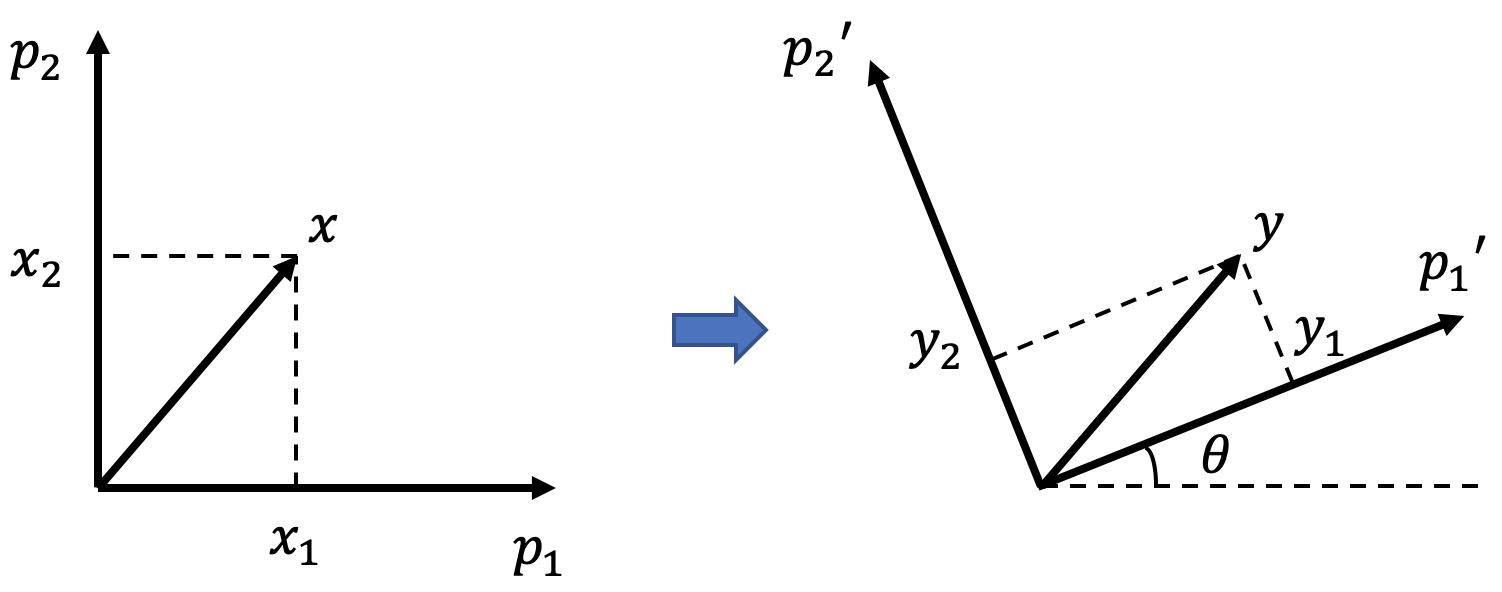图 3：坐标轴旋转

• 在二维空间 $\mathbb R^2$ 中，我们可以通过 正交矩阵 来描述一个角度为 $\theta$ 的旋转：

$\Gamma = \begin{pmatrix}\cos(\theta) & \sin(\theta) \\ -\sin(\theta) & \cos(\theta)\end{pmatrix}$

具体来说，如果坐标轴的原始集合 以原点为中心逆时针旋转 一个角度 $\theta$，则原始坐标轴系统中坐标为 $x$ 的点的新坐标 $y$ 为：

$y=\Gamma x$

如果是 顺时针旋转，则为：

$y=\Gamma^{\mathrm T}x$

• 更一般地，在向量 $x$ 左边乘以一个 正交矩阵 $\Gamma$ 在几何上对应于坐标轴系统的一次旋转。

## 2. 均值、协方差和相关系数

### 2.1 均值

• 一个随机向量 $X=(X_1,\dots,X_p)^{\mathrm T}$ 的 均值 (Mean) $\mu\in \mathbb R^p$ 被定义为：

$\mu=\begin{pmatrix}\mu_1 \\ \vdots \\ \mu_p\end{pmatrix}=\begin{pmatrix}E(X_1) \\ \vdots \\ E(X_p)\end{pmatrix}$

• 在实践中，我们无法计直接对 $\mu$ 进行计算 (因为我们无法观测到总体)，但是我们可以根据来自一个样本 $X_1,\dots,X_n$ 的 样本均值 来估计它：

$\overline X=\begin{pmatrix}\overline X_1 \\ \vdots \\ \overline X_p\end{pmatrix}$

其中，对于 $j=1,\dots,p$，

$\overline X_j = \dfrac{1}{n}\sum_{i=1}^{n}X_{ij}$

是第 $j$ 个变量 $X_j$ 的样本均值。

• 回顾矩阵 $\mathcal X$ 的表示形式：

$\mathcal X=\begin{pmatrix} X_{11} & \cdots & X_{1p} \\ X_{21} & \cdots & X_{2p} \\ & \vdots & \\ X_{n1} & \cdots & X_{np} \end{pmatrix}$

以及一个长度为 $n$ 的列向量 $1_n=(1,\dots,1)^{\mathrm T}$。我们可以将 $\overline X$ 表示为 矩阵形式

$\overline X=n^{-1}\mathcal X^{\mathrm T}1_n$

### 2.2 协方差矩阵

• 两个随机变量 $X$ 和 $Y$ 之间的 协方差 (Covariance) $\sigma_{XY}$ 是这两个随机变量之间的 线性依赖性 (linear dependence) 的一种度量：

$\sigma_{XY}=\mathrm{Cov}(X,Y)=E(XY)-E(X)E(Y)$
• $\sigma_{XX}=\mathrm{Var}(X)$
• 如果 $X$ 和 $Y$ 彼此独立，则 $\sigma_{XY}=0$
• 但是反之则不然，$\sigma_{XY}=0$ 并不意味着 $X$ 和 $Y$ 彼此独立 (有可能存在非线性依赖)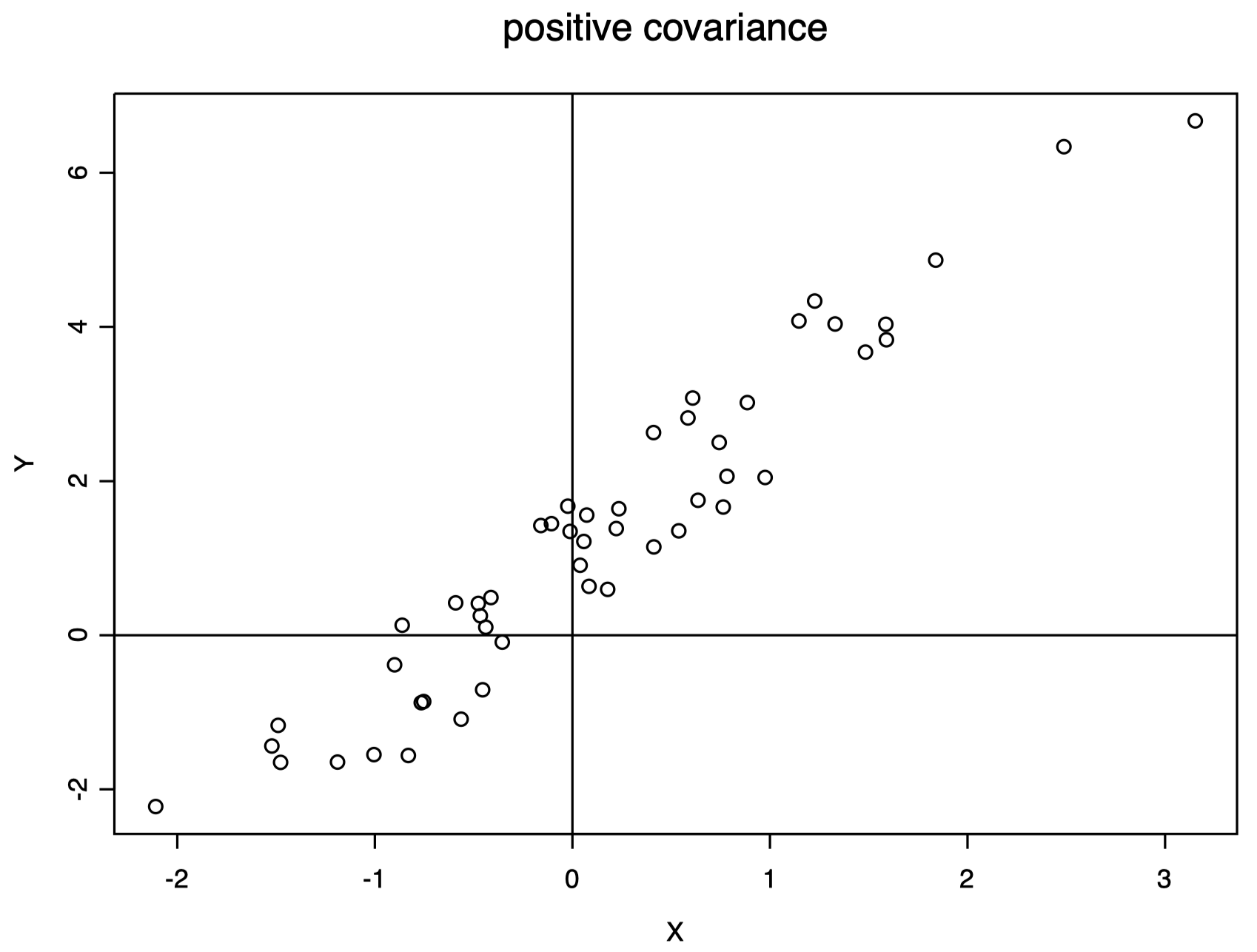图 4：协方差为正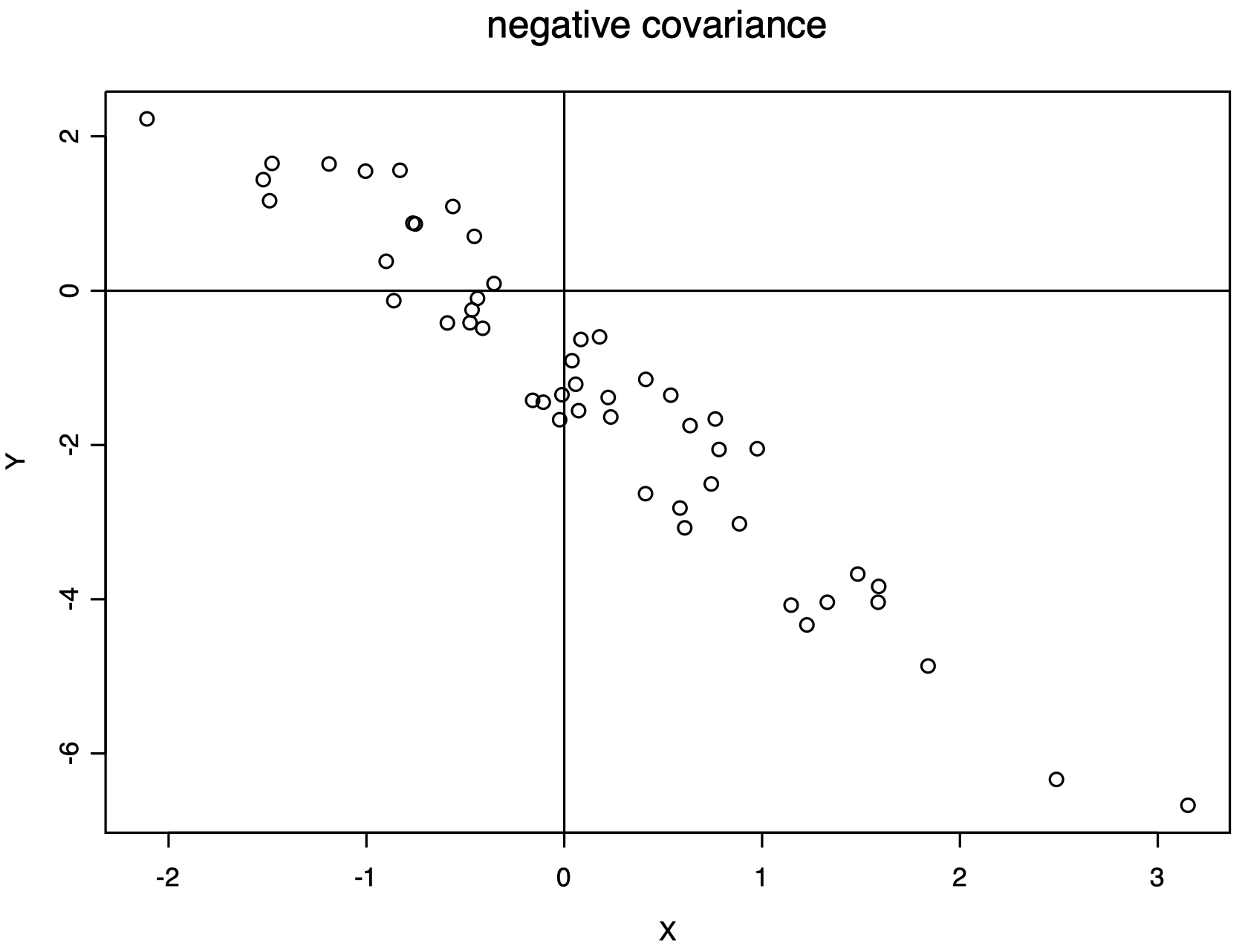图 5：协方差为负

• 如果 $X=(X_1,\dots,X_p)^{\mathrm T}$ 是一个 $p$ 维随机向量，我们可以计算每一对 $X_i$ 和 $X_j$ 之间的协方差，并将所有结果汇总到一个 $p\times p$ 的 协方差矩阵 $\Sigma$ 中：

$\Sigma = \begin{pmatrix}\sigma_{X_1X_1} & \cdots & \sigma_{X_1X_p} \\ & \vdots & \\ \sigma_{X_pX_1} & \cdots & \sigma_{X_pX_p}\end{pmatrix}= \begin{pmatrix}\sigma_{11} & \cdots & \sigma_{1p} \\ & \vdots & \\ \sigma_{p1} & \cdots & \sigma_{pp}\end{pmatrix}$
• 我们可以将 $X$ 的协方差记为 $\Sigma_X$
• 注意 $\Sigma$ 是对称的：$\Sigma=\Sigma^{\mathrm T}$
• 矩阵表示 下，我们可以将其写为：

$\Sigma=E\{(X-\mu)(X-\mu)^{\mathrm T}\}$

其中，$X$ 和 $\mu$ 被写成 $p$ 维列向量。

• 在实践中，我们无法直接计算 $\Sigma$，但是我们可以根据来自一个样本 $X_1,\dots,X_n$ 的 样本协方差矩阵 来估计它：

$\mathcal S=\begin{pmatrix}s_{X_1X_1} & \cdots & s_{X_1X_p} \\ & \vdots & \\ s_{X_pX_1} & \cdots & s_{X_pX_p}\end{pmatrix}= \begin{pmatrix}s_{11} & \cdots & s_{1p} \\ & \vdots & \\ s_{p1} & \cdots & s_{pp}\end{pmatrix}$

其中，对于 $j,k=1,\dots,p$，

$s_{X_jX_k}=s_{jk}=\dfrac{1}{n-1}\sum_{i=1}^{n}(X_{ij}-\overline X_j)(X_{ik}-\overline X_k)$

是变量 $X_j$ 和 $X_k$ 之间的样本协方差。

• 注意 $\mathcal S$ 是 对称的 ($\mathcal S=\mathcal S^{\mathrm T}$) 和 半正定的

• 矩阵表示 下，我们可以将其写为：

$\mathcal S=\dfrac{1}{n-1}\mathcal X^{\mathrm T}\mathcal X-\dfrac{n}{n-1}\overline X \,\overline X^{\mathrm T}$

其中，$\mathcal X$ 是 $n\times p$ 的数据矩阵，$\overline X$ 被写成 $p$ 维列向量。

• 提示：请始终检查矩阵尺寸是否兼容 (即矩阵乘积是否有意义等)。

### 2.3 相关系数矩阵

• 协方差矩阵的问题：它不是 单位不变的 (unit invariant)。如果我们更改变量的度量单位，则协方差也会随之更改。

• 相关系数 (Correlation) 是单位不变的线性相关性的量度。

• 一个 $p$ 维随机向量 $X=(X_1,\dots,X_p)^{\mathrm T}$ 的 相关系数矩阵 $\mathcal P$ 是一个 $p\times p$ 的矩阵，其定义如下：

$\mathcal P=\begin{pmatrix}1 & \rho_{12} & \cdots & \rho_{1p} \\ \rho_{21} & 1 & \cdots & \rho_{2p} \\ & \vdots & & \\ \rho_{p1} & \rho_{p2} & \cdots & 1\end{pmatrix}$

其中，

$\rho_{ij}=\dfrac{\sigma_{ij}}{\sqrt{\sigma_{ii}\sigma_{jj}}}$

是变量 $X_i$ 和 $X_j$ 之间的相关系数。

• 我们总是有 $-1\le \rho_{ij} \le 1$
• $\rho_{ij}$ 是变量 $X_i$ 和 $X_j$ 之间 线性关系 的一种度量
• $|\rho_{ij}|=1$ 意味着完美线性关系
• $\rho_{ij}=0$ 意味着不存在线性关系，但是这并不能说明两者是独立的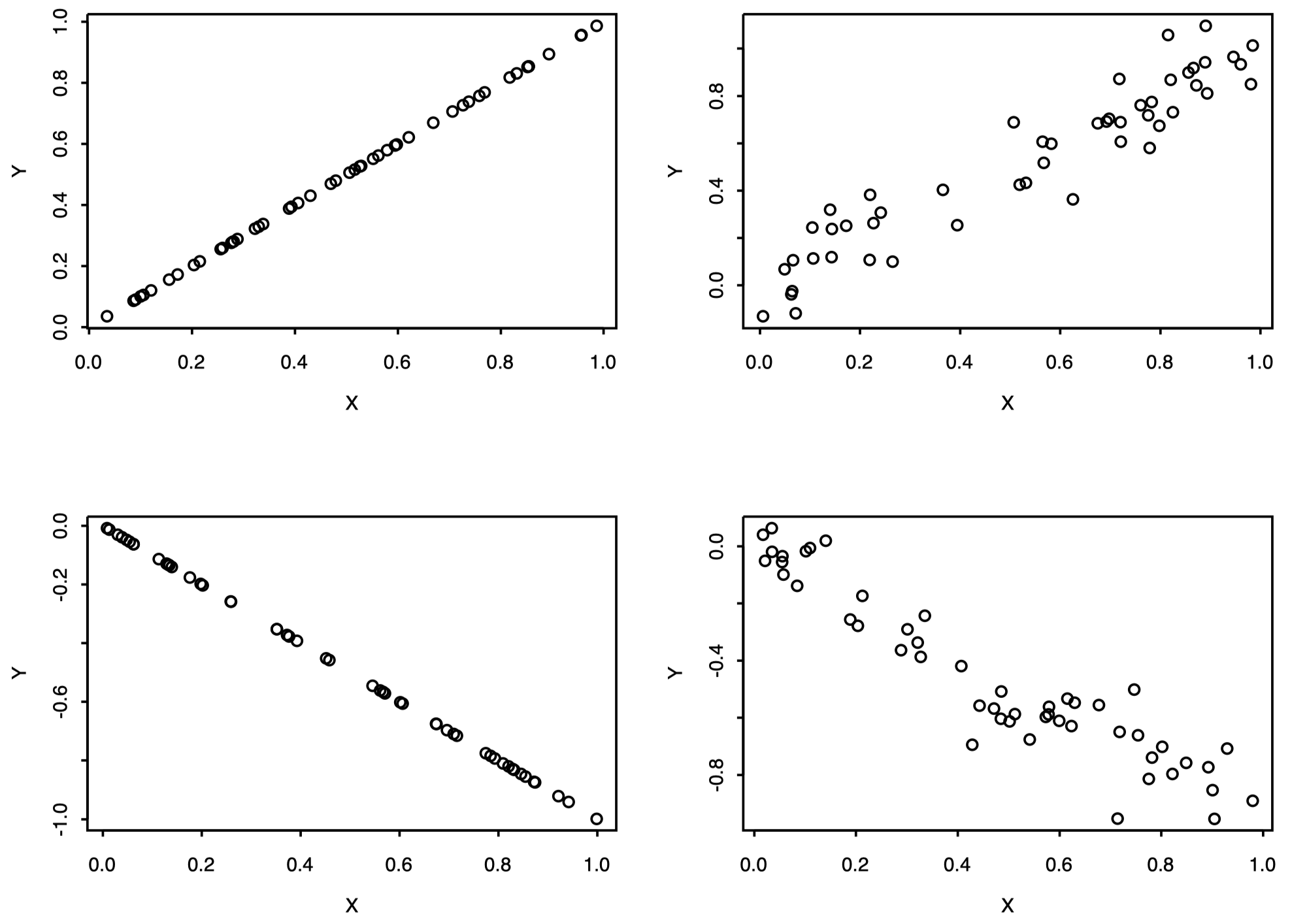图 6：线性关系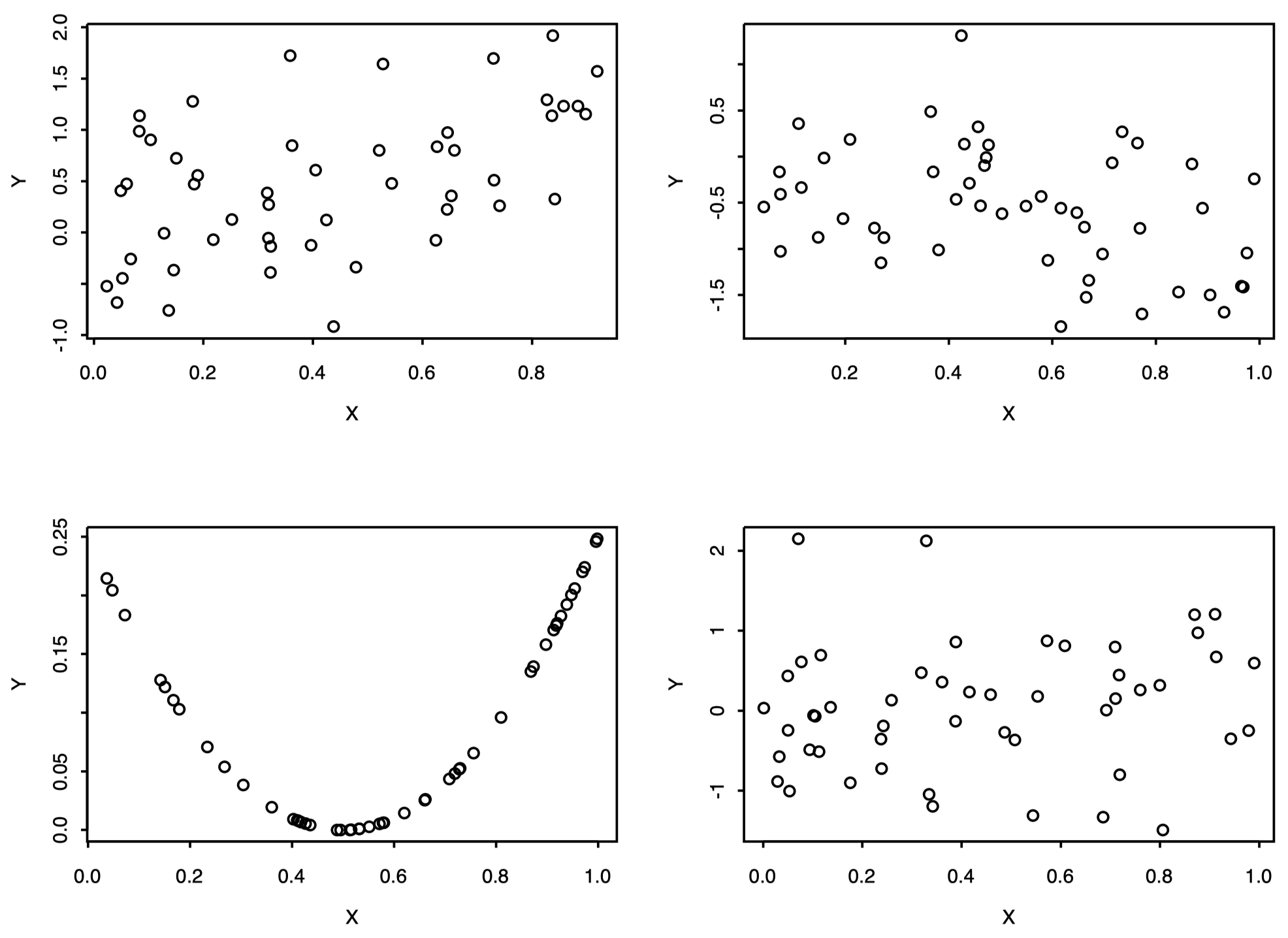图 7：非线性关系

• 矩阵表示 下，我们可以将其写为：

$\mathcal P=\mathcal D^{-1/2}\Sigma \mathcal D^{-1/2}$

其中，$\Sigma$ 是 $p\times p$ 的协方差矩阵，而

$\mathcal D=\mathrm{diag}(\sigma_{11},\dots,\sigma_{pp})$

是 $p\times p$ 的对角矩阵，其对角线上的元素就是每个变量的方差。

• 在实践中，我们无法直接计算 $\mathcal P$，但是我们可以根据来自一个样本 $X_1,\dots,X_n$ 的 样本相关系数矩阵 来估计它：

$\mathcal R = \begin{pmatrix}r_{11} & \cdots & r_{1p} \\ & \vdots & \\ r_{p1} & \cdots & r_{pp}\end{pmatrix}$

其中，对于 $j,k=1,\dots,p$，

$r_{jk}=\dfrac{s_{jk}}{\sqrt{s_{jj}s_{kk}}}$

是变量 $X_i$ 和 $X_j$ 之间的样本相关系数。

• 矩阵表示 下，我们可以将其写为：

$\mathcal R=\mathcal D^{-1/2}\mathcal S \mathcal D^{-1/2}$

其中，$\mathcal S$ 是 $p\times p$ 的样本协方差矩阵，而

$\mathcal D=\mathrm{diag}(s_{11},\dots,s_{pp})$

是 $p\times p$ 的对角矩阵，其对角线上的元素就是每个变量的样本方差。

### 2.4 线性变换

$Y=\mathcal A X + b$

\begin{align} E(Y) &= \mathcal A E(X) +b \\[2ex] \overline Y &= \mathcal A \overline X +b \\[2ex] \Sigma_Y &= \mathcal A \Sigma_X \mathcal A^{\mathrm T} \\[2ex] \mathcal S_Y &= \mathcal A \mathcal S_X \mathcal A^{\mathrm T} \end{align}
• 提示：为了知道转置矩阵放置的位置，请始终检查矩阵尺寸是否兼容。本作品采用知识共享署名-非商业性使用-相同方式共享 4.0 国际许可协议进行许可。 欢迎转载，并请注明来自：YEY 的博客 同时保持文章内容的完整和以上声明信息！